# Left Rotate a Binary Tree in javascript

How did I end up here? I ask myself. I'm sitting in my couch in front of the TV, but it's not turned on. The clock is way past bedtime and I'm scribbling binary trees, transforming them, while drinking coffee.

It started with implementing a toy compiler on my spare time. I managed to parse a set of expressions that would add integers together.

``````1 + 2 + 3 + 4 + 5
``````

Addition is as simple as it gets, because `a + b = b + a`. I knew it was generating an expression tree, but I didn't really care what it looked like.

And this evening I thought I should just add subtraction to mix it up.

``````1 - 2 + 3 - 4 + 5
``````

The implementation was easy enough and the result was `5`. Now, wait a minute. That doesn't seem right. The result should be `3` right? Suddenly I needed to start caring about that expression tree.

The reason for it to evaluate to 5 would be that the expression tree looks like this.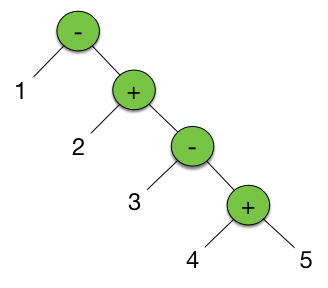``````1 - (2 + (3 - (4 + 5))) = 5
``````

This is not how you do this calculation. Instead you should calculate it from left to right, like this.

``````(((1 - 2) + 3) - 4) + 5 = 3
``````

Going back to the expression tree we would need something that looks like this.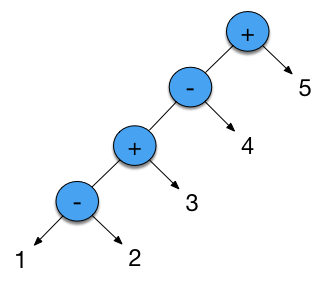In order to accomplish this you can do something called left rotation of the binary tree and there is an excellent article on Wikipedia on how to do this. The following image describes this very well.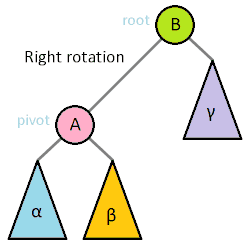This is easy enough when you have a complete tree that you want to rotate, the problem is that my tree was built from bottom up. This is very common, so I needed a way of rotating the tree while building it.

Let's first take a look at how the tree is built.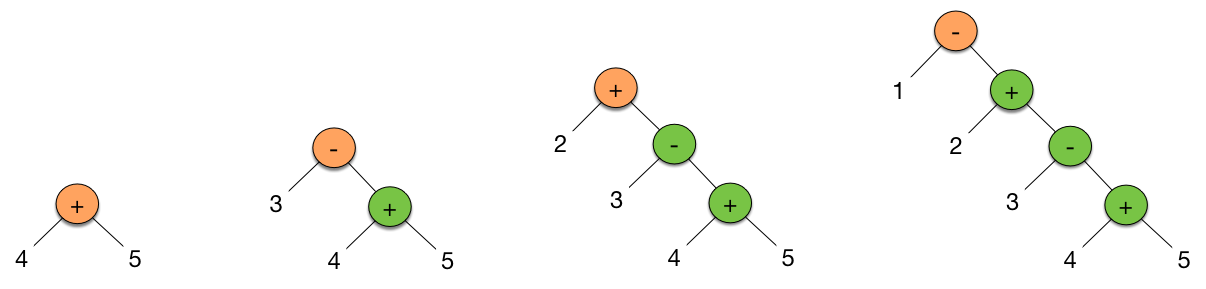If we're to visualize this in javascript it would look like this.

```new Expression('-',
new Value(1),
new Expression('+',
new Value(2),
new Expression('-',
new Value(3),
new Expression('+',
new Value(4),
new Value(5))
)
)
);```

What I would like to do in the `Expression` constructor, is to rotate the tree to the left. This is not that hard to do if you think of it.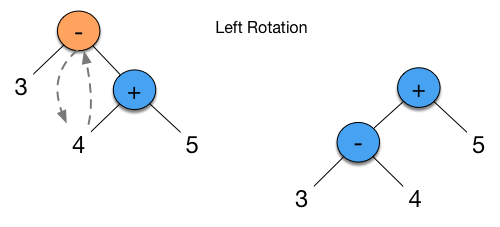Doing this in javascript is surprisingly easy.

```function leftRotate(node) {
var rightNode = node.right;
```// right is a node and not a leaf
if (rightNode.nodeType === &#39;node&#39;) {
node.right = rightNode.left;
rightNode.left = node;
return leftRotate(rightNode);
}

return rightNode;
```
};```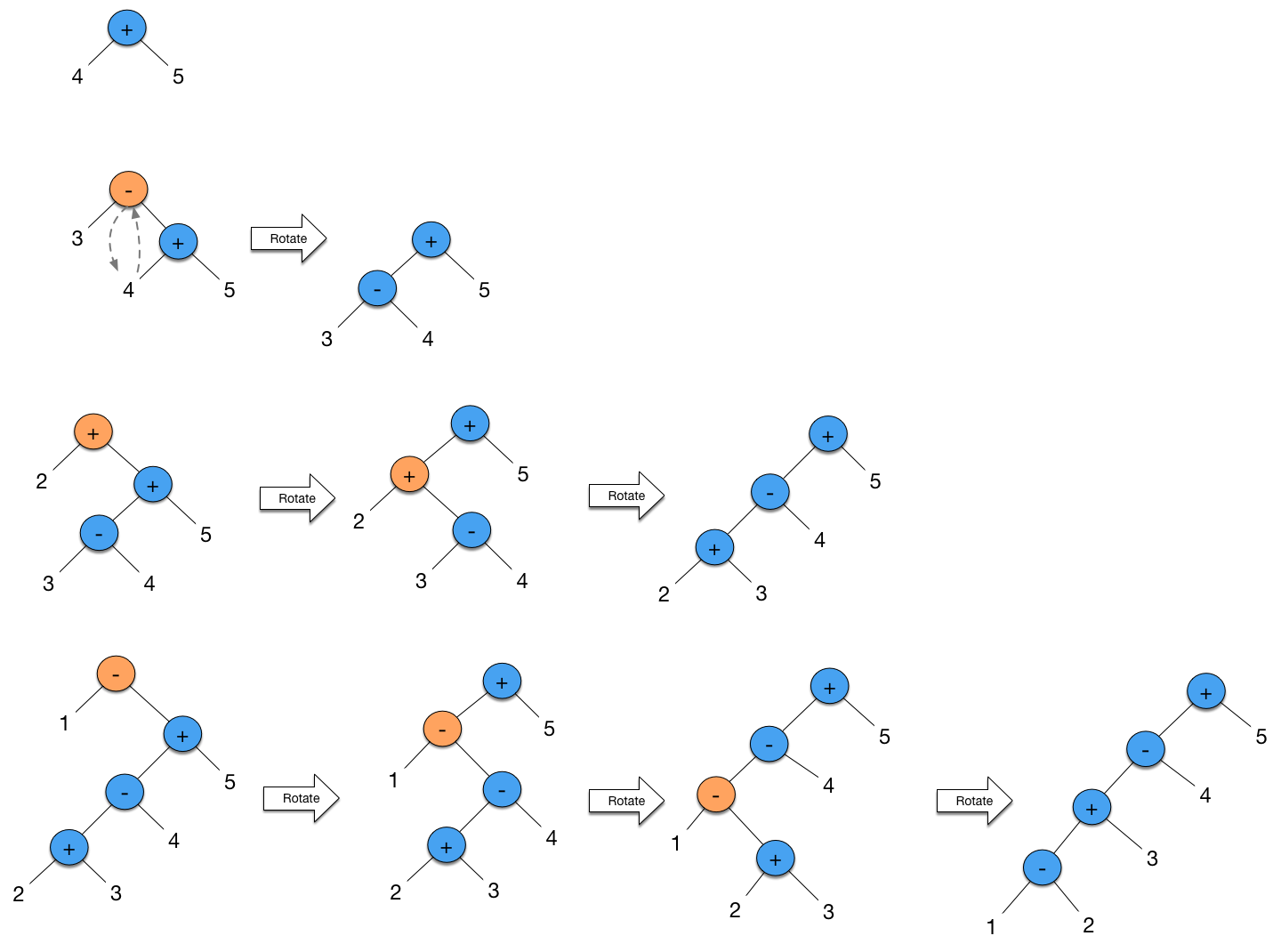For every node that is added, it needs to be left rotated until there is no more `right` nodes to rotate with.

This function works very well when working the tree from outside the Expression, but what I try to do, is to rotate the tree in the constructor as the tree is being created.

```var Expression = function(content, left, right) {
this.content = content;
this.left = left;
this.right = right;
```// ERROR: this is not assignable
this = leftRotate(this);
```
};```

Since the constructor cannot assign this, there is no way to do this as clean as before. We need to rewrite `leftRotate` so it copy the references and content instead of moving the entire node down the tree.

```function leftRotate(node) {
var rightNode = node.right;
```// right is a node and not a leaf
if (rightNode.nodeType === &#39;node&#39;) {
// copy node content
var content = node.content;
node.content = rightNode.content;
rightNode.content = content;

// left rotation
node.right = rightNode.right;
rightNode.right = rightNode.left;
rightNode.left = node.left;
node.left = rightNode;

leftRotate(rightNode);
}
```
};

var Expression = function(content, left, right) {
this.content = content;
this.left = left;
this.right = right;
```// left rotate this node down the tree
leftRotate(this);
```
};```

This is not as pure as the first solution, but this actually works inside the Expression constructor by simply mutating the binary tree in a left rotation.